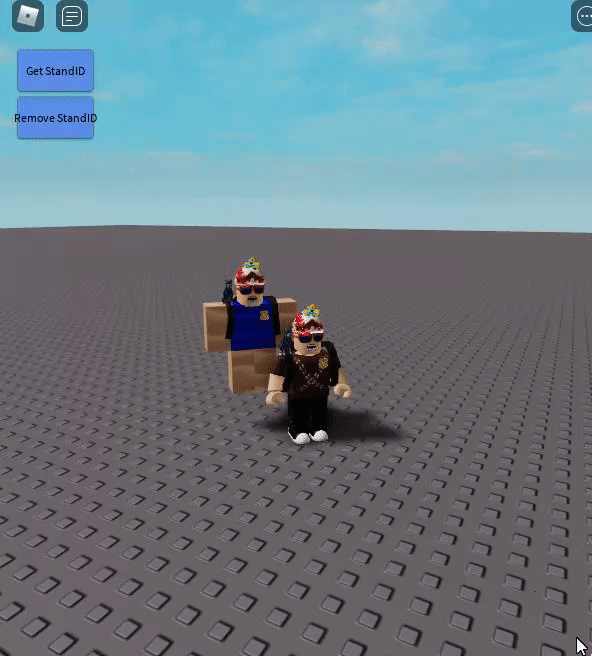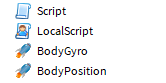# Part Move CFrame Script Lag

Hello, i need help for reduct (if is possible) the lag cosed by my codeI have try to use “RunService” but it just made it worse

So, for explaint, This is a NPC whout humanoid so is not relly a npc and on the “NPC” on HumanoidRootPart i have thisThe Script and LocalScript is same. This is the script

``````--local Run = game:GetService("RunService")
local Huma = script.Parent.Parent.Parent:WaitForChild("HumanoidRootPart")
local Model = script.Parent
--Run.Heartbeat:Connect(function()
--script.Parent.CFrame = script.Parent.Parent.Parent:WaitForChild("HumanoidRootPart").CFrame * CFrame.new(3,2,2)
--wait()
--local p = Huma.CFrame * Vector3.new(2,2,2)
--Model.BodyGyro.CFrame = Huma.CFrame
--Model.BodyPosition.Position = Vector3.new(p.x, p.y, p.z)
--end)

while true do
wait()
local p = Huma.CFrame * Vector3.new(2,2,2)
Model.BodyGyro.CFrame = Huma.CFrame
Model.BodyPosition.Position = Vector3.new(p.x, p.y, p.z)
end
``````

There isn’t much you can do, here’s how I would optimize it.

``````local Huma = script.Parent.Parent.Parent:WaitForChild("HumanoidRootPart")
local Model = script.Parent

while wait() do
local p = Huma.CFrame * Vector3.new(2,2,2)
Model.BodyGyro.CFrame = Huma.CFrame
Model.BodyPosition.Position = p.p
end
``````

@lynnlo what is the difference between

``````	Model.BodyPosition.Position = p.p
``````

and

``````	Model.BodyPosition.Position = Vector3.new(p.x, p.y, p.z)
``````

?
Edit
I try it and is not work/ give me errorOh sorry I thought p was a CFrame.
You can just use.
`Model.BodyPosition.Position = p`
And it’ll work.

1 Like

@lynnlo so, this ?

``````local Huma = script.Parent.Parent.Parent:WaitForChild("HumanoidRootPart")
local Model = script.Parent

while wait() do
local p = Huma.CFrame * Vector3.new(2,2,2)
Model.BodyGyro.CFrame = Huma.CFrame
Model.BodyPosition.Position = p
end
``````

Try using RenderStepped. This runs before the frame is rendered.

https://developer.roblox.com/en-us/api-reference/event/RunService/RenderStepped

1 Like